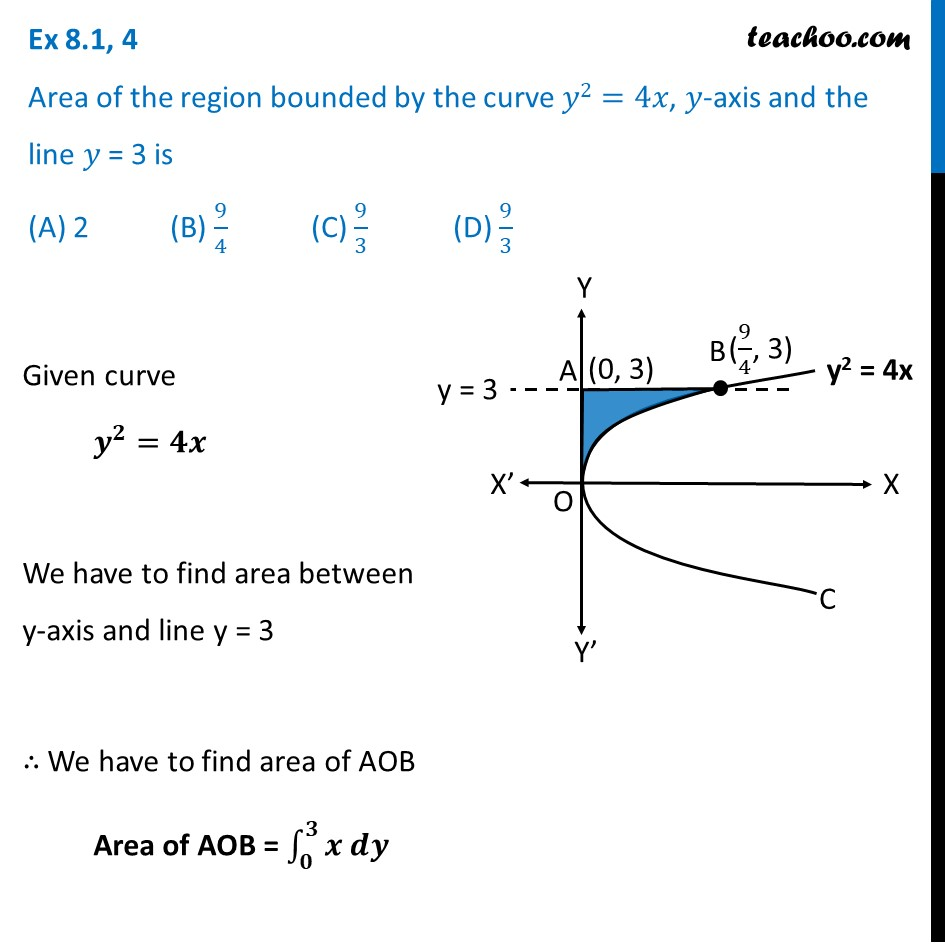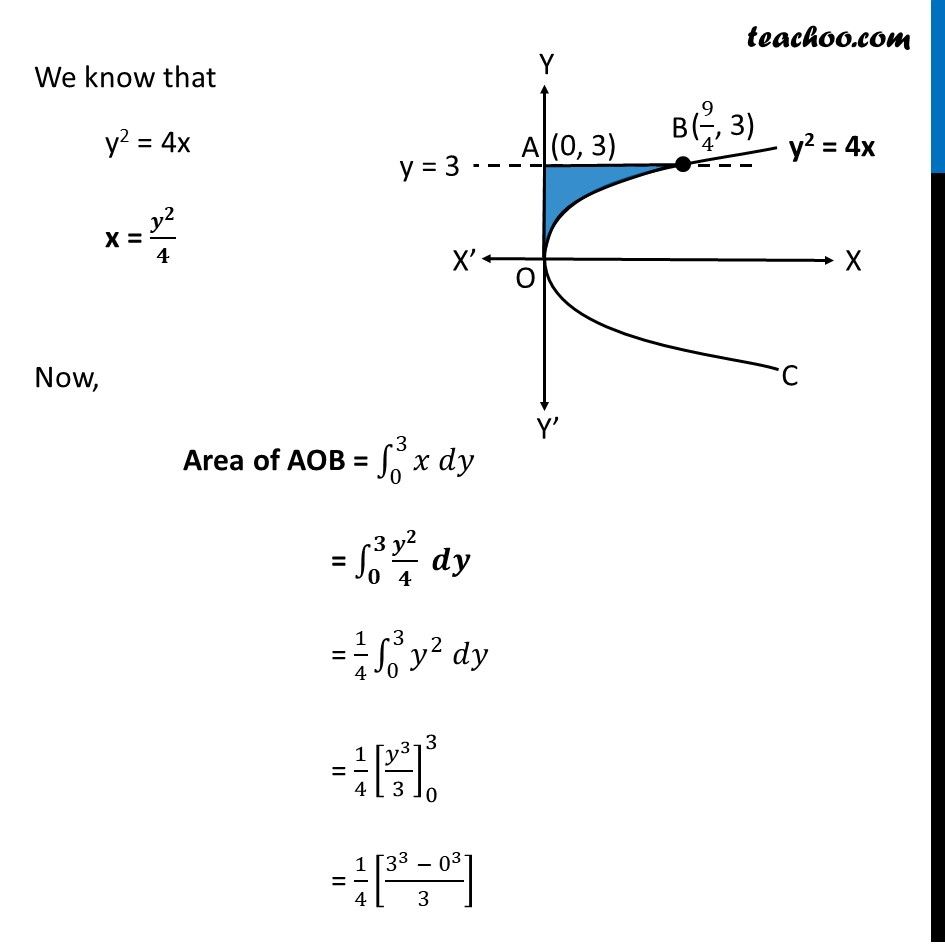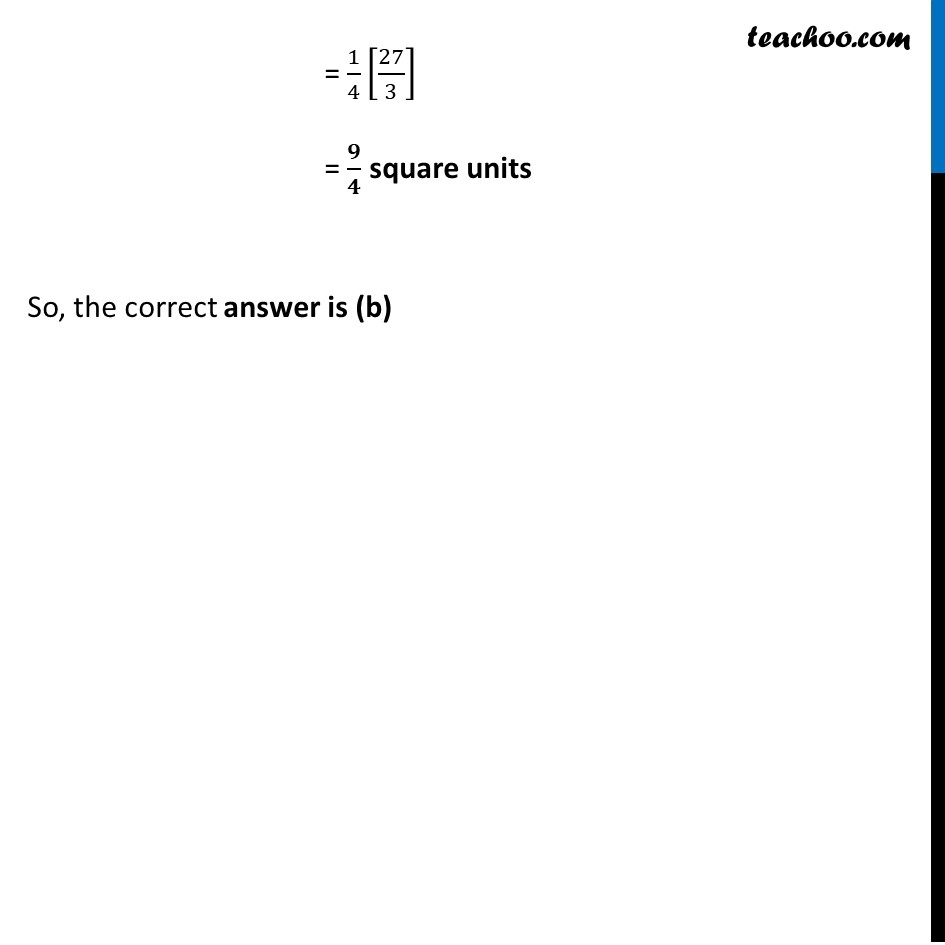Ex 8.1

Chapter 8 Class 12 Application of Integrals
Serial order wiseLearn in your speed, with individual attention - Teachoo Maths 1-on-1 Class

### Transcript

Ex 8.1, 4 Area of the region bounded by the curve 𝑦2=4𝑥, 𝑦-axis and the line 𝑦 = 3 is (A) 2 (B) 9/4 (C) 9/3 (D) 9/3Given curve 𝒚^𝟐=𝟒𝒙 We have to find area between y-axis and line y = 3 ∴ We have to find area of AOB Area of AOB = ∫1_𝟎^𝟑▒〖𝒙 𝒅𝒚〗 We know that y2 = 4x x = 𝒚^𝟐/𝟒 Now, Area of AOB = ∫1_0^3▒〖𝑥 𝑑𝑦〗 = ∫1_𝟎^𝟑▒〖𝒚^𝟐/𝟒 𝒅𝒚〗 = 1/4 ∫1_0^3▒〖𝑦^2 𝑑𝑦〗 = 1/4 [𝑦^3/3]_0^3 = 1/4 [(3^3 − 0^3)/3] = 1/4 [27/3] = 𝟗/𝟒 square units So, the correct answer is (b)